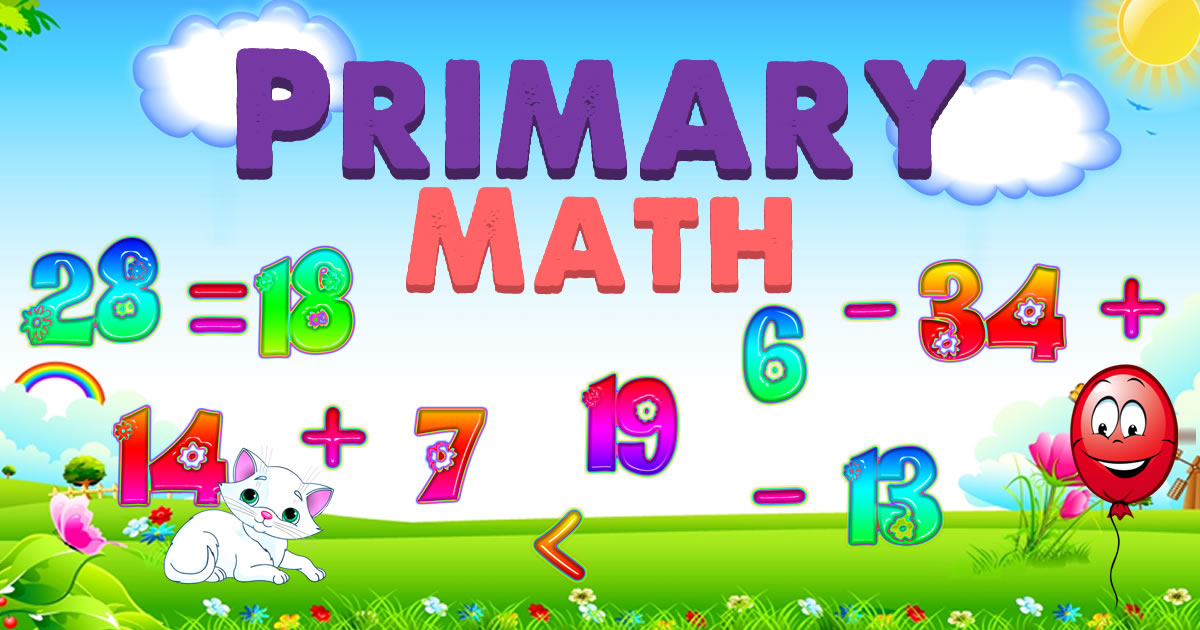# Primary Math

In game Primary Math, you are given mainly 40 levels through that you have to go. In each level, the difficulty of the game increases as there is much harder numbers are given to be calculated. Either you are given the addition of numbers or given the subtraction of numbers. In the game, you just have to give it the game and put the right answer at the right place. Have fun.
nan%
Deel met vrienden
Opmerkingen over Primary Math